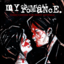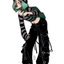MathematicsOpenStudy (anonymous):

Verify that parallelogram ABCD with vertices A(–5, –1), B(–9, 6), C(–1, 5), and D(3, –2) is a rhombus by showing that it is a parallelogram with perpendicular diagonals.OpenStudy (jamesj):

So first you need to show it is a parallelogram. You do that by showing opposite sides are parallel. You do that by showing that they have the same slopes. (or if you know vectors by showing the sides point in the same direction). Next you need to show the diagonals are perpendicular. Do you that by showing the slopes of the diagonals have the characteristic of perpendicular lines: i.e., the product of their slopes is -1. But the thing you should do before either of those steps, call it "Step Zero" is draw a diagram. Draw a diagram of the figure by sketching out the location of the four vertices and the sides between them.OpenStudy (anonymous):

Thanks. Can you answer another question for me?OpenStudy (jamesj):

I'm about to go out, but post it on the left and I will or someone will get to it, I'm sure.

Latest Questionsshalong: What was John Calhounu2019s major objection to high tariffs?
11 minutes ago 0 Replies 0 Medalshayhay6: Give an example of an equation of a line with an undefined slope. What type of li
46 minutes ago 1 Reply 0 Medalshayhay6: What is the equation of a line that passes through the point (5, -3) and has a sl
56 minutes ago 1 Reply 0 Medalspiperkoebel: Does anyone know the coordinates of two points on the line y = u22122?
59 minutes ago 1 Reply 0 Medalslolokrat: anyone good with microeconomics that can help me find out where i went wrong on m
12 hours ago 0 Replies 0 MedalsAsleepAndUnafraid21: URGENT PLZ HELP NOW Part C: Find the distance from B to E and from P to E. Show your work.
13 hours ago 49 Replies 5 MedalsKachirikutoka: How many solutions does the following equation have? |3x + 12| = 18 a No solution
18 hours ago 1 Reply 1 MedalAsleepAndUnafraid21: In the figure below, segment DE is parallel to segment BC and segment EF is parallel to AB: The figure shows triangle ABC with segments DE and DF.
15 hours ago 11 Replies 0 MedalsAsleepAndUnafraid21: In the figure below, segment DE is parallel to segment BC and segment EF is parallel to AB: The figure shows triangle ABC with segments DE and DF.
19 hours ago 1 Reply 0 Medalslakeawilliams: What is the solution to StartFraction 5 over 6 EndFraction x minus one-third grea
20 hours ago 1 Reply 0 Medals